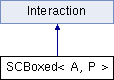ParM  parm A molecular dynamics library
SCBoxed< A, P > Class Template Reference

#include <interaction.hpp>

Inheritance diagram for SCBoxed< A, P >:## Public Member Functions

SCBoxed (sptr< AtomVec > atomv, sptr< SCBox > box)

void add (A atm)

flt energy (Box &box)
Potential energy due to this Interaction. More...

flt pressure (Box &box)
Partial pressure due to this Interaction. More...

void set_forces (Box &box)

flt set_forces_get_pressure (Box &box)
Set forces (Atom.f) and return $$P = \sum_{\left<i,j \right>} \vec r_{ij} \cdot \vec F_{ij}$$ at the same time (see pressure()). More...

vector< A > & atom_list ()Public Member Functions inherited from Interaction
virtual Matrix stress (Box &box)
The force-moment tensor for the current simulation: More...

virtual ~Interaction ()

## Protected Attributes

sptr< SCBoxbox

sptr< AtomVecatoms

vector< A > group

## Constructor & Destructor Documentation

template<class A , class P >
 SCBoxed< A, P >::SCBoxed ( sptr< AtomVec > atomv, sptr< SCBox > box )
inline

## Member Function Documentation

template<class A , class P >
 void SCBoxed< A, P >::add ( A atm )
inline
template<class A , class P >
 vector& SCBoxed< A, P >::atom_list ( )
inline
template<class A , class P >
 flt SCBoxed< A, P >::energy ( Box & box )
virtual

Potential energy due to this Interaction.

Implements Interaction.

template<class A , class P >
 flt SCBoxed< A, P >::pressure ( Box & box )
virtual

Partial pressure due to this Interaction.

$$P = \sum_{\left<i,j \right>} \vec r_{ij} \cdot \vec F_{ij}$$, or equivalently $$P = \sum_i \vec r_i \cdot \vec F_i$$

Note that the full pressure involves all interactions and temperature, and needs to be normalized by $$\frac{1}{dV}$$ where $$d$$ is the number of dimensions and $$V$$ is the volume.

Implements Interaction.

template<class A , class P >
 void SCBoxed< A, P >::set_forces ( Box & box )
virtual

Implements Interaction.

template<class A , class P >
 flt SCBoxed< A, P >::set_forces_get_pressure ( Box & box )
virtual

Set forces (Atom.f) and return $$P = \sum_{\left<i,j \right>} \vec r_{ij} \cdot \vec F_{ij}$$ at the same time (see pressure()).

Reimplemented from Interaction.

## Member Data Documentation

template<class A , class P >
 sptr SCBoxed< A, P >::atoms
protected
template<class A , class P >
 sptr SCBoxed< A, P >::box
protected
template<class A , class P >
 vector SCBoxed< A, P >::group
protected

The documentation for this class was generated from the following file: